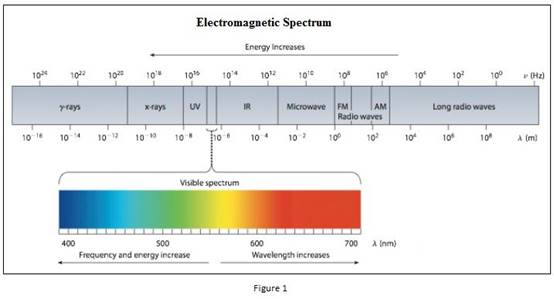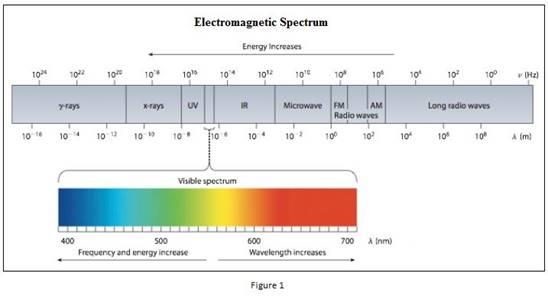# Among green light and red light, the colour having the higher energy photons should be identified. Concept introduction: Electromagnetic radiations are a type of energy surrounding us. They are of different types like radio waves, IR, UV, X-ray etc. The red light and green light lies in the visible light where it is between 400 nm and 700 nm . Planck’s equation, E = hν = hc λ where, E = energy h = Planck's constant ν = frequency The energy increases as the wavelength of the light decrease. Also the energy increases as the frequency of the light increases. The frequency of the light is inversely proportional to its wavelength. ν = c λ where, c = speed of light ν = frequency λ = wavelength### Chemistry & Chemical Reactivity

9th Edition
John C. Kotz + 3 others
Publisher: Cengage Learning
ISBN: 9781133949640### Chemistry & Chemical Reactivity

9th Edition
John C. Kotz + 3 others
Publisher: Cengage Learning
ISBN: 9781133949640

#### Solutions

Chapter 6, Problem 55GQ

(a)

Interpretation Introduction

## Interpretation: Among green light and red light, the colour having the higher energy photons should be identified.Concept introduction: Electromagnetic radiations are a type of energy surrounding us. They are of different types like radio waves, IR, UV, X-ray etc.The red light and green light lies in the visible light where it is between 400 nm and 700 nm. Planck’s equation,  E = hν = hcλwhere, E = energyh = Planck's constantν = frequencyThe energy increases as the wavelength of the light decrease. Also the energy increases as the frequency of the light increases. The frequency of the light is inversely proportional to its wavelength.  ν = cλwhere, c = speed of lightν = frequencyλ = wavelength

(b)

Interpretation Introduction

### Interpretation: Among green light and red light, the colour having 500 nm and 680 nm wavelength should be identified.Concept introduction: Electromagnetic radiations are a type of energy surrounding us. They are of different types like radio waves, IR, UV, X-ray etc.The red light and green light lies in the visible light where it is between 400 nm and 700 nm.

(c)

Interpretation Introduction

### Want to see the full answer?

Check out a sample textbook solution.

### Want to see this answer and more?

Experts are waiting 24/7 to provide step-by-step solutions in as fast as 30 minutes!*

*Response times may vary by subject and question complexity. Median response time is 34 minutes for paid subscribers and may be longer for promotional offers.